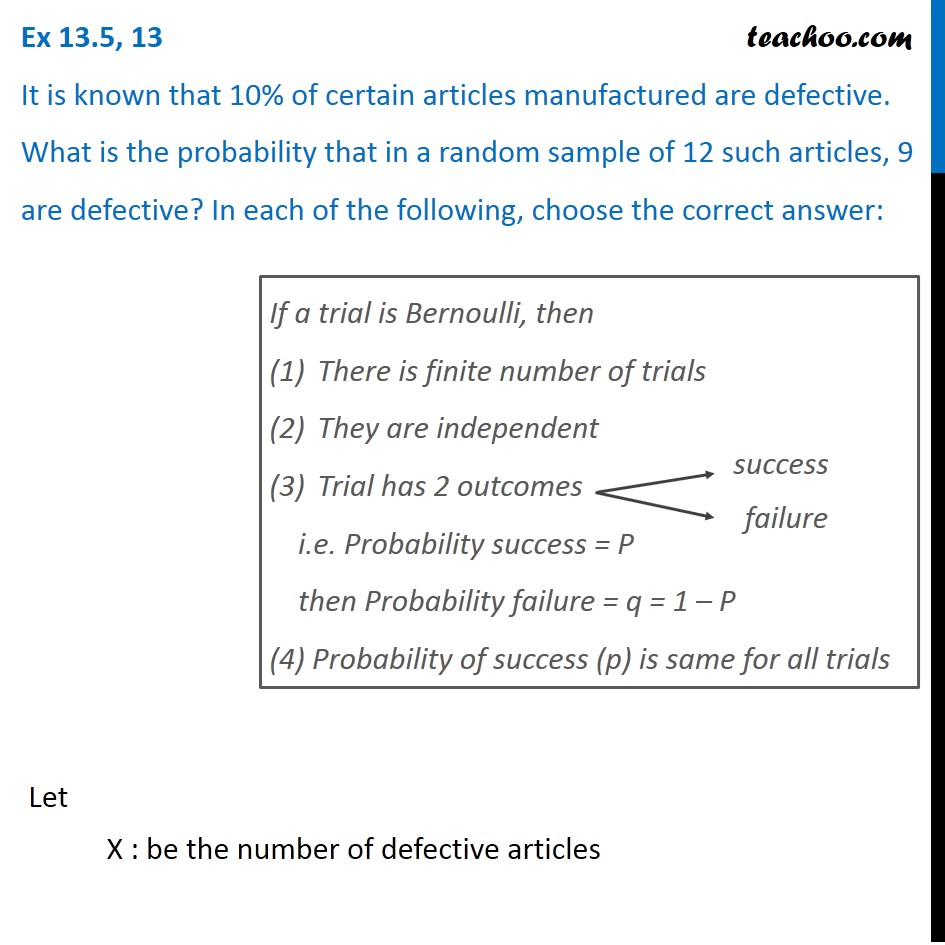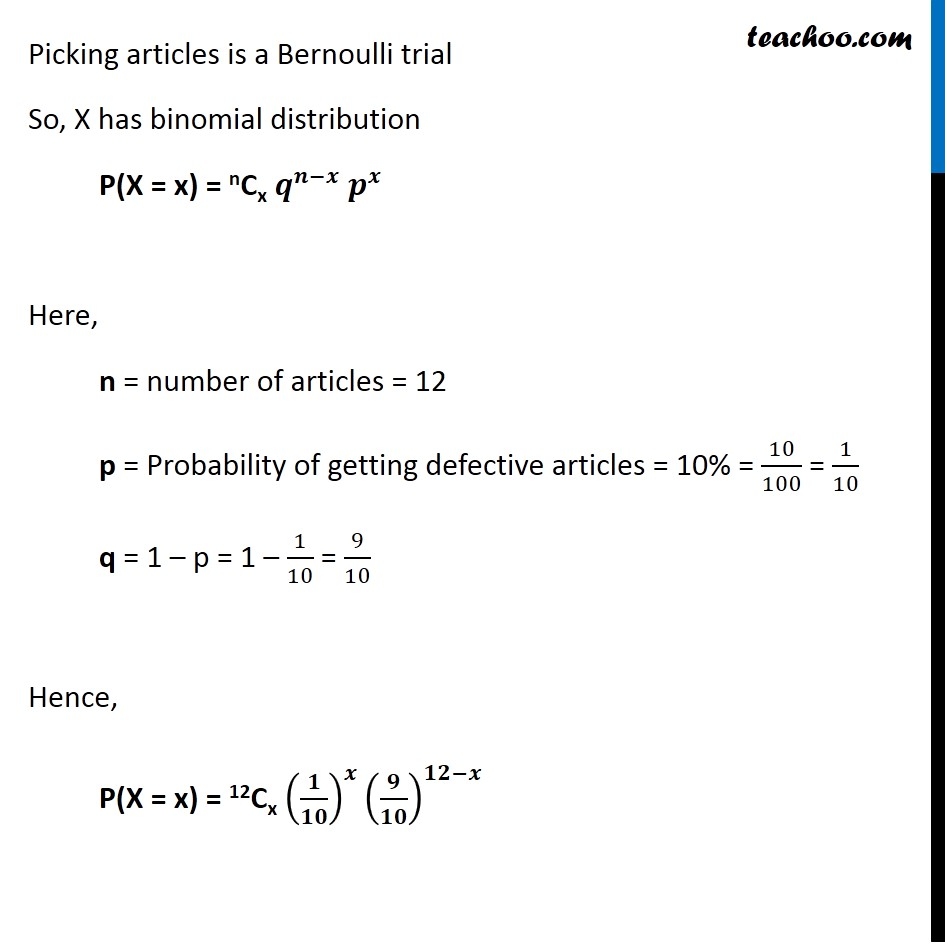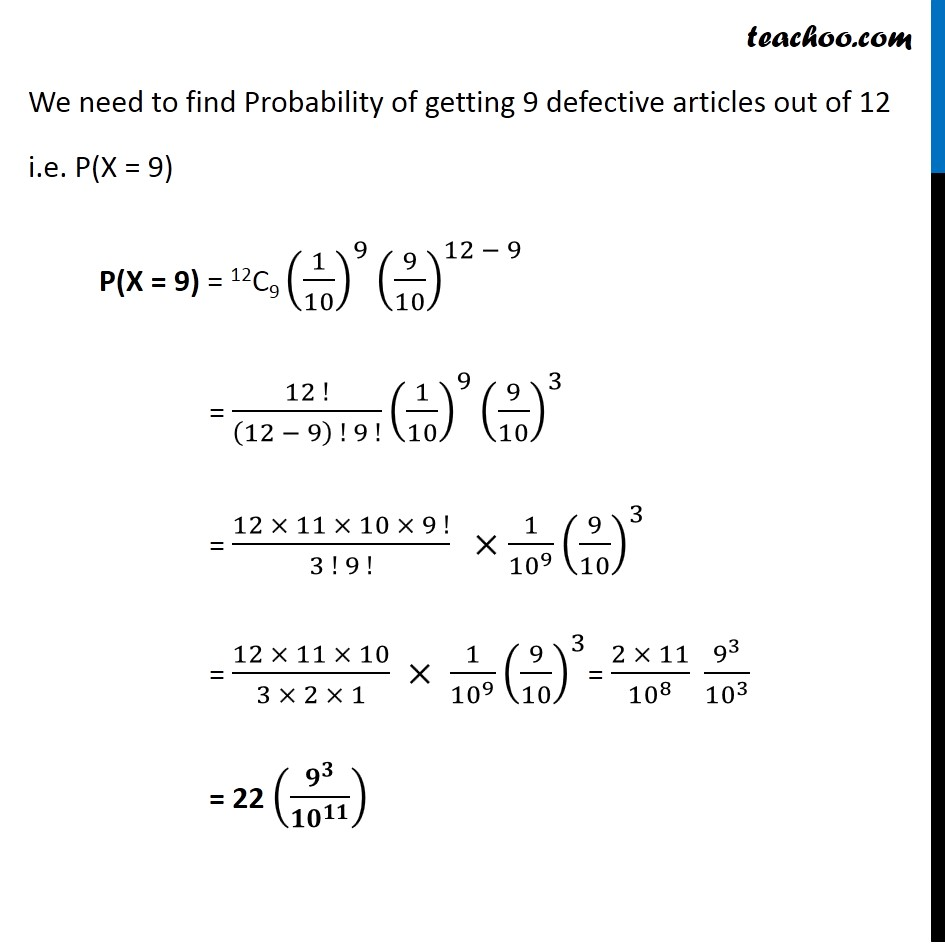Chapter 13 Class 12 Probability

Class 12
Important Questions for exams Class 12Learn in your speed, with individual attention - Teachoo Maths 1-on-1 Class

### Transcript

Question 13 It is known that 10% of certain articles manufactured are defective. What is the probability that in a random sample of 12 such articles, 9 are defective? In each of the following, choose the correct answer:vIf a trial is Bernoulli, then There is finite number of trials They are independent Trial has 2 outcomes i.e. Probability success = P then Probability failure = q = 1 – P (4) Probability of success (p) is same for all trials Let X : be the number of defective articles Picking articles is a Bernoulli trial So, X has binomial distribution P(X = x) = nCx 𝒒^(𝒏−𝒙) 𝒑^𝒙 Here, n = number of articles = 12 p = Probability of getting defective articles = 10% = 10/100 = 1/10 q = 1 – p = 1 – 1/10 = 9/10 Hence, P(X = x) = 12Cx (𝟏/𝟏𝟎)^𝒙 (𝟗/𝟏𝟎)^(𝟏𝟐−𝒙) Picking articles is a Bernoulli trial So, X has binomial distribution P(X = x) = nCx 𝒒^(𝒏−𝒙) 𝒑^𝒙 Here, n = number of articles = 12 p = Probability of getting defective articles = 10% = 10/100 = 1/10 q = 1 – p = 1 – 1/10 = 9/10 Hence, P(X = x) = 12Cx (𝟏/𝟏𝟎)^𝒙 (𝟗/𝟏𝟎)^(𝟏𝟐−𝒙) We need to find Probability of getting 9 defective articles out of 12 i.e. P(X = 9) P(X = 9) = 12C9 (1/10)^9 (9/10)^(12 − 9) = (12 !)/((12 − 9) ! 9 !) (1/10)^9 (9/10)^3 = (12 × 11 × 10 × 9 !)/(3 ! 9 !) \ ×1/〖10〗^9 (9/10)^3 = (12 × 11 × 10)/(3 × 2 × 1) × 1/〖10〗^9 (9/10)^3= (2 × 11)/〖10〗^8 9^3/〖10〗^3 = 22 (𝟗^𝟑/〖𝟏𝟎〗^𝟏𝟏 )